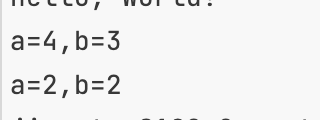# 一元运算符

• i++、i--
先赋值后运算
• ++i、--i
先运算后赋值
``````int a=3;
int b=a++;
Console.WriteLine(\$"a={a},b={b}");
a=3;
b=--a;
Console.WriteLine(\$"a={a},b={b}");
``````+、-、*、/、%

=、+=、-=、*=、/=

## 逻辑运算符

&、｜、^(异或运算符，只有一个为true则为true。相同为false、相反为true)

&&、||

## 移位运算符

<<、>>其能够更快地执行某些运算

## 其他运算符

• nameof以字符串的形式返还变量、类型或成员的短路名称（可用于输出异常消息）
• sizeof返回简单类型的字节大小（确定内存大小效率）
``````int age=47;
Console.WriteLine(nameof(age));
Console.WriteLine(sizeof(int));
``````

# 流程控制

## if

C7.0增加了if语句可以将is关键字与局部变量声明结合，判断其是否为某种类型，如果是则临时赋值，并且可以在语句块中使用该变量，使用更安全

``````if (true)
{

}
else if (false)
{

}
object o = "3";
if (o is int a)
{
Console.WriteLine(true);
Console.WriteLine(a);
}
else
{
Console.WriteLine(false);
Console.WriteLine(o);
}
``````

## swith语句

swith语句对应每个case的内容可以有：

• break 退出swith
• goto case 关键字
• 没有语句
• return 退出当前函数
• default 未匹配
应避免使用goto跳转到另一个case

## swith在C#7之后可以匹配模式

``````List<string> list = new List<string>();
switch (list)
{
case List<string>:
Console.WriteLine("List");
break;
}
``````

# 迭代语句

## while

• while和do while
``````while (true)
{

}
do
{

} while (true);
``````

## for循环

• fori循环
``````for (int i = 1; i < 10; i++)
{

}
``````
• foreach循环
foreach循环需要对象实现迭代器，内部获得的对象不能修改原对象的值
``````foreach (string s1 in list)
{

}
``````

# 类型转换

## 强制类型转换

• 隐式
安全，自动执行（小->大）
• 显式
不安全可能会丢失信息，手动执行（大->小）
使用()强转
``````int a=10;
double b=a;//小转大，隐式

long c=20;//大转小，显式
a=(int)c;
``````

## System.Convert类型转换

### 基本类型

• System.Convert可以转换所有基本类型、字符串、日期和时间
``````var int32 = System.Convert.ToInt32(b);
``````

### 转换数字

• 可以四舍五入或者自定义取整规则

### 二进制、Base64字符串互相转换

• 将二进制对象转换为Base64字符串
Convert.ToBase64String
• 将Base64字符串转换为二进制对象
Convert.FromBase64String

### 日期、时间和字符串相互转换

• 转换为字符串
ToString
• 转换为日期、时间
Parse()，如果不能转换则会抛出异常可以用TryParse()方法先进行验证，如果可以转换则为true，否则返回false